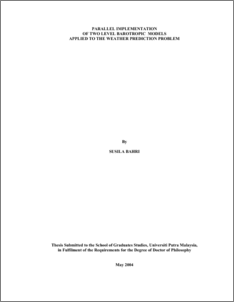# Parallel Implementation of Two Level Barotropic Models Applied to the Weather Prediction Problem

## Citation

Bahri, Susila (2004) Parallel Implementation of Two Level Barotropic Models Applied to the Weather Prediction Problem. PhD thesis, Universiti Putra Malaysia.

## Abstract

Forecasting model for short range weather prediction that is used here is the two level Barotropic models. This model is used because it can give approximation of air conditions covering higher isobar levels. By using finite difference approximation, quasigeostrophic vorticity equations and thermodynamic equations are used to develop forecasting equations for each level. The forecasting equation for determining the geopotential heights can be solved by using suitable lateral conditions. In relation to the conformal map, the quasigeostrophic equations are modified by using a formula. These equations are then transformed into finite difference equation. This can be achieved by using grids chosen from the mesh scale. Then, the system of equations is solved using Gauss Seidel method. To process the data collected from British Atmospheric Data Centre (BADC), the sequential programs in row and columnwise fashions are developed and implemented. Then the parallel algorithms are constructed and run using the Beowulf Cluster machine. The numerical results based on the sequential and parallel algorithms for both row and columnwise fashions are presented and compared to determine their performance.Preview
PDF
549609_T_FS_2004_4.pdfView Item